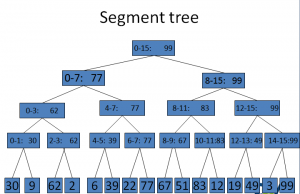## Data Structures: Segment Trees

Data Structures are a way to store data in such a way that we can operate on them efficiently. The way we are organizing our data matters a lot. If we manage our data in the right way, we can reduce the running time of our operations on the data by a lot.

This talk is about such a data structure known as segment tree.

First lets see why we need segment trees. I demonstrate it using a problem statement:

You are given an array of n integers (a to a[n-1]).

You have to answer m queries.
In each query, you are given two integers, l and r ( 0<=l,r<=n-1 ). Your job is to print the largest number in the range a[l], a[l+1], a[l+2],…., a[r-1], a[r].
n is in the order of 10^6
m is in the order of 10^5.

Iterate from l to r and keep track of the maximum number found.
Eg. For query 2 7, look at all the numbers from 2 till 7. Easy to find the max number this way.
In form of a ‘for’ loop:
int max=array[l];
for(i=l;i<=r;i++)

{

if(array[i]>max)

max=array[i];
}
Though very simple to understand, this method is very time consuming.
For each query, it does (r-l+1) operations. For n=1000000, and a query where l=0 and r=999999, 10^6 iterations will take place. As I mentioned earlier, there can be 10^5 queries like this. So, the total operations required to answer all queries will be 10^5 X 10^6= 10^11.

For n=1000000 and m=100000, this method will take 120 seconds to run. And this is a lot!

The better way to solve this problem is using segment tree.

The principle of segment tree is:
If we have two arrays
{3,5,2} and {6,22,1,6,10}.
And the maximum integer of these arrays is m1=5 and m2=22 respectively. Then, the maximum of the array obtained by combining both these array {3,5,2,6,22,1,6,10} is MAX(m1,m2)=MAX(5,22)=22.
i.e. We don’t need to look at the whole combined array again. We can deduce the maximum of the whole array just by looking at the maximum of the individual arrays

Segment tree for the array {30,9,62,2,6,39,22,77,67,51,83,12,19,49,3,99} is as follows:Using this segment tree, we can reduce the number of nodes that we have to look at to answer a query. For eg.
for the query 4 15, we have to look at the two nodes labelled 4-7 and 8-15. And the answer for the query 4 15 will be the MAX of the value of the two abovementioned nodes i.e. MAX(77,99)=99.
For the query 4 13, we will look at the three nodes labelled 4-7 and 8-11 and 12-13. And similarly find that the answer to the query is MAX(77,83,49)=83.
You may observe that you never have to look at more then 2 X log2(n) nodes for answering any query.
So, for n=1000000, m=100000, the number of operations required to answer all queries is 2 X log2(1000000) X 100000 = 4 X 10^6 operations.
And indeed, this algorithm runs on the same input in only around 0.35 seconds!

Click the following to download the .pdf on segment trees used during the Talk.

CEV TALK SEGMENT TREES

CEV - Handout